# C++ Data Types with Examples

The data are the values used in the program. Data can be of many types, for example, character, integer, real, string, etc. Anything enclosed in single quotes represents character data in C++. Numbers without fractions represent integer data. Numbers with fractions represent real data, and anything enclosed in double quotes represents a string.

So, C++, like any other language, provides ways and facilities to handle different types of data by providing data types.

Data types are used to identify the type of data and the operations that go with it. C++ data types are of the following two types:

Now, in brief, let's go over the details of the above two data type categories in C++. But, before we get into the details of the two data type categories mentioned above, here is a list of other topics that fall under the category of "data types" and are also covered in this article. This list is provided for your convenience so that you can navigate it at your leisure. Otherwise, you will learn about each "data type" topic in this article one by one.

## C++ Fundamental Data Types

Fundamental data types are those that are not composed of other data types. There are the following five fundamental data types:

Please note: The "int" data type represents integers, the "char" data type represents characters, the "float" data type represents floating-point numbers, the "double" data type represents double precision floating-point numbers, the "bool" data type refers to boolean type values (true or false), and the "void" data type represents an empty set of values.

### int type in C++

Integers are whole numbers such as 4, 56, -234, 0, etc. They have no fractional parts. Integers are represented in C++ by the int data type.

An identifier declared as an "int" cannot have a fractional part. Let's take an example program.

```#include<iostream>
using namespace std;
int main()
{
int a, b, res;
cout<<"Enter the two numbers: ";
cin>>a>>b;
res = a+b;
cout<<"\nSum = "<<res;
cout<<endl;

return 0;
}```

Here is a sample run of the above C++ program: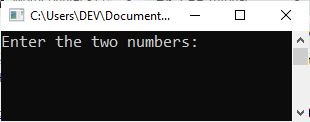Now supply the input, say 210, and hit the "ENTER" key, and then 40 and hit the "ENTER" key again to see the following output: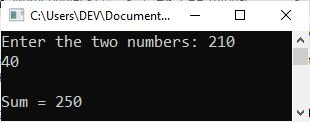As already stated, an "int" type value cannot have a fractional part. For example:

```#include<iostream>
using namespace std;
int main()
{
int num;
cout<<"Enter a number: ";
cin>>num;
cout<<"\nYou entered: "<<num;
cout<<endl;

return 0;
}```

The following snapshot shows the sample run of the above C++ example with user input "123.658."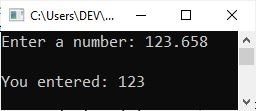The fractional part will be removed automatically if you try to initialize a number with a fractional part to a variable of the "int" type. You need to use the "float" type in that case.

### char type in C++

Characters can store any member of the C++ implementation's basic character set. If a character from this set is stored in a character variable, its value is equivalent to the integer code of that character. An identifier declared as "char" becomes a character variable.

```#include<iostream>
using namespace std;
int main()
{
char myChar;
cout<<"Enter a character: ";
cin>>myChar;
cout<<"\nYou entered: "<<myChar;
cout<<endl;

return 0;
}```

The sample run of the above C++ example should be:

```Enter a character: c

You entered: c```

### float type in C++

A floating-point number is any number that contains a fractional component. A number in floating-point format might look something like 3.14159. A floating-point number, as opposed to an integer number, can be identified by the presence of the decimal point. The number 12 is considered to be an integer, whereas the number 12.0 is considered to be a floating-point number. This is written out using standard decimal notation (fractional form). Exponentiation is another form that floating-point numbers can be written in. For instance, 147.9101 would be represented by the fraction 1.479101E02.

An identifier that is declared to be "float" transforms into a variable that can store floating-point numbers and becomes a floating-point variable. Real numbers are typically denoted by a fractional component and are employed for measurable quantities such as distance, area, temperature, and so on. Floating point variables are used to represent real numbers.

Create an example of the "float" type for yourself. Come on, it's your exercise. Create a program that receives marks obtained in six subjects and prints the average and percentage marks.

#### int vs. float in C++

In comparison to integers, floating-point numbers have two advantages. For starters, they can represent values between integers. Second, they are capable of representing a much broader range of values. However, floating-point numbers have one disadvantage as well. In general, floating-point operations are slower than integer operations.

### double type in C++

In addition, the handling of floating-point numbers can be done with the data type double. However, it is still considered to be a separate data type due to the fact that it requires twice as much memory as the float type and can store floating-point numbers with a significantly greater range and precision (significant numbers after the decimal point).

It is used in situations where the precision offered by type float is insufficient.

### bool type in C++

The "bool" type in C++ is used when we need to store the following two values:

• true
• false

Please note: Don't use any type of quote while initializing a value (true or false) to a "bool" type variable. For example:

`bool x = true;`

The term "true" refers to "1," whereas "false" refers to "0." For example:

```#include<iostream>
using namespace std;
int main()
{
bool x = true, y = false;
cout<<x<<endl;
cout<<y<<endl;

return 0;
}```

The output of the above example for the "bool" type should be: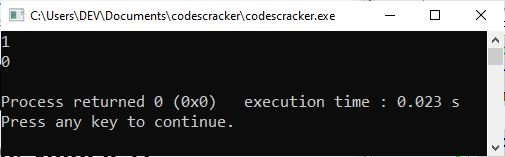### void type in C++

The void type specifies an empty set of values. It is used as the return type for functions that do not return a value. No object of type "void" may be declared.

The "void" is essentially a keyword that can be used in place of a data type to represent the absence of data wherever you would normally put that data type. As an illustration, the statement:

`void codescracker();`

indicates that the function "codescracker()" does not return any value.

## C++ Data Types Size and Range

The size and range of these data types vary with each processor type and with the implementation of the C++ compiler. Most of the time, a character takes up one byte and an integer takes up two bytes, but you can't assume this if you want your programs to work on as many computers as possible.

Data Type Storage Size (in Bytes) Range
char 1 -127 to 127
unsigned char 1 0 to 255
signed char 1 -127 to 127
int 2 or 4 -32,767 to 32,767
unsigned int 2 or 4 0 to 65,535
signed int 2 or 4 -32,767 to 32,767
short int 2 -32,767 to 32,767
unsigned short int 2 0 to 65,535
signed short int 2 -32,767 to 32,767
long int 4 -2,147,483,647 to 2,147,483,647
signed long int 4 -2,147,483,647 to 2,147,483,647
unsigned long int 4 0 to 4,294,967,295
float 4 1E-37 to 1E+37 (six digits of precision)
double 8 1E-37 to 1E+37 (ten digits of precision)
long double 10 1E-37 to 1E+37 (ten digits of precision)
bool 1 true or false
void 0 empty

A byte is made up of 8 bits, with a bit being the smallest unit of memory. 1 bit can represent two distinct binary number combinations (0 and 1), i.e., 21, 2 bits can represent four distinct binary number combinations (00, 01, 10, 11,) i.e., 22, and so on. As a result, a single byte (8 bits) can represent 28 = 256 different combinations. A two-byte unit, for example, can represent 65536 (216) different values.

To determine the actual size of a variable on your platform, use the "sizeof()" method. As an example:

```#include<iostream>
using namespace std;
int main()
{
cout<<"\"int\" = "<<sizeof(int);
cout<<"\n\"unsigned int\" = "<<sizeof(unsigned int);
cout<<"\n\"signed int\" = "<<sizeof(signed int);
cout<<endl;

return 0;
}```

My system has a 64-bit architecture, and the following is the output of the above C++ example on my platform, using the "Code::Blocks" IDE: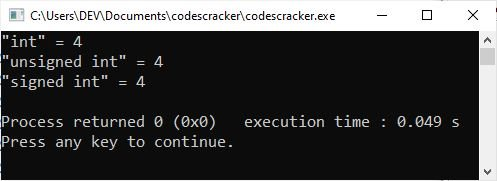Please note: The \n is used to insert a line break on the output console, whereas \" is used to output a double quote.

## Data Type Assignment Rules in C++

The following guidelines should be followed when assigning data types:

• When you are certain that a variable contains only integers, i.e., no decimal points, you should assign it the short, int, or long data type. Choose the "long" format when the integers to be processed are large.
• When it is necessary to work with fractional numbers, also known as numbers that contain decimals, assign the float or double data type. The "double" data type should be used whenever possible because it eliminates the possibility of rounding-off errors.
• If the variable will always contain one character of data, assign it the char data type. This indicates that there is only one letter (or character).

## C++ Derived Data Types

Using the declaration operators, you can take the basic data types and turn them into other types. Here are some of the most important data types derived from C++ fundamental types.

• Array
• Function
• Pointer
• Reference

Some other derived types are: class, structure, union, enum, and typedef. These are also called "user-defined types." You will learn all about these derived types in the coming articles or tutorials.

#### More Examples

Here are some C++ programs that you might enjoy:

C++ Quiz

« Previous Tutorial Next Tutorial »